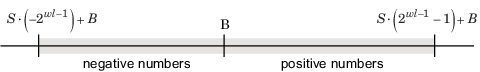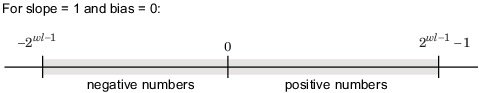## 范围和精度

### 范围#### 范围限制

Fixed-Point Designer™ 软件允许您对溢出进行饱和绕回处理。饱和将正溢出表示为正在使用的范围中的最大正数，将负溢出表示为正在使用的范围中的最大负数。绕回使用模算术将溢出转换回数据类型的可表示范围。

### 精度

#### 精度限制

`$V\approx \stackrel{˜}{V}=SQ+B={2}^{-2}Q+32,$`

`$\begin{array}{l}\stackrel{˜}{V}={2}^{-2}\left(13\right)+32=35.25,\\ \stackrel{˜}{V}={2}^{-2}\left(14\right)+32=35.50.\end{array}$`

`$|\stackrel{˜}{V}-V|=0.125=\frac{S}{2}=\frac{F{2}^{E}}{2}.$`

`$|\stackrel{˜}{V}-V|`

### 定点数据类型参数

`fixdt(0,ws,0)`

0

${2}^{ws}-1$

`1`

`fixdt(1,ws,0)`

$-{2}^{ws-1}$

${2}^{ws-1}-1$

`1`

`fixdt(0,ws,fl)`

0

$\left({2}^{ws}-1\right){2}^{-fl}$

${2}^{-fl}$

`fixdt(1,ws,fl)`

$-{2}^{ws-1-fl}$

$\left({2}^{ws-1}-1\right){2}^{-fl}$

${2}^{-fl}$

`fixdt(0,ws,s,b)`

`b`

$s\left({2}^{ws}-1\right)+b$

s

`fixdt(1,ws,s,b)`

$-s\left({2}^{ws-1}\right)+b$

$s\left({2}^{ws-1}-1\right)+b$

s

s = 斜率，b = 偏置，ws = 字长，fl = 小数长度

21

2.0

-256, 254

0, 510

20

1.0

-128, 127

0, 255

2-1

0.5

-64, 63.5

0, 127.5

2-2

0.25

-32, 31.75

0, 63.75

2-3

0.125

-16, 15.875

0, 31.875

2-4

0.0625

-8, 7.9375

0, 15.9375

2-5

0.03125

-4, 3.96875

0, 7.96875

2-6

0.015625

-2, 1.984375

0, 3.984375

2-7

0.0078125

-1, 0.9921875

0, 1.9921875

2-8

0.00390625

-0.5, 0.49609375

0, 0.99609375

### 8 位定点数据类型的范围和精度 - 斜率和偏置定标

1

1.25

-159, 159.75

1, 319.75

1

0.625

-79, 80.375

1, 160.375

1

0.3125

-39, 40.6875

1, 80.6875

1

0.15625

-19, 20.84375

1, 40.84375

1

0.078125

-9, 10.921875

1, 20.921875

1

0.0390625

-4, 5.9609375

1, 10.9609375

1

0.01953125

-1.5, 3.48046875

1, 5.98046875

1

0.009765625

-0.25, 2.240234375

1, 3.490234375

1

0.0048828125

0.375, 1.6201171875

1, 2.2451171875